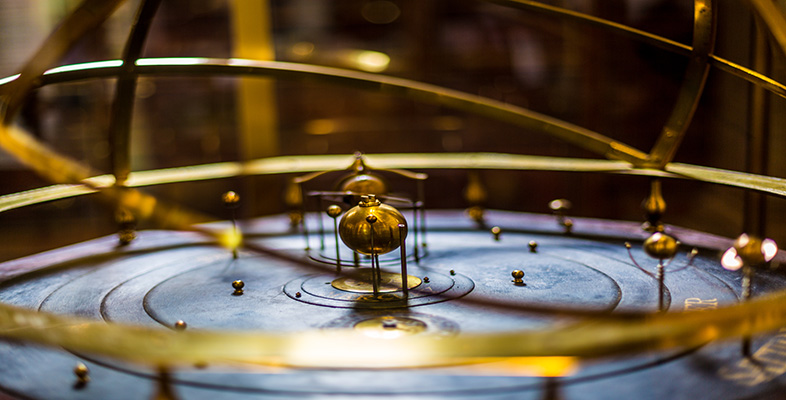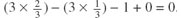Science, Maths & Technology

### Become an OU studentThe evolving Universe

Start this free course now. Just create an account and sign in. Enrol and complete the course for a free statement of participation or digital badge if available.

# 1.5 The quark-lepton era

Time: l0−11s to 10−5s

Temperature: 3 × 1015 K to 3 × 1012 K

Energy: 1000 GeV to 1 GeV

During the time interval 10−32 s to 10−11 s, i.e. for the 10−11 seconds or so after inflation, nothing new happened in the Universe! It merely carried on expanding and cooling, but no new processes took place. The desert (as it is known) - came to an end when the Universe reached a temperature of about 3 × 1015 K, and this is where the next stage in our history begins. At this point the mean energy per particle was around 1000 GeV and the electromagnetic and weak interactions became distinct (Figure 1). The energies corresponding to this transition are becoming attainable in experiments here on Earth. So it could be argued that all particle reactions that models propose after the first 10−11 s of the history of the Universe are directly testable in Earth-based laboratories.

By 10−11 s after the Big Bang, the X bosons had long since decayed in reactions like those shown in Equation 2, but the temperature of the Universe was still too high for the familiar baryons (protons and neutrons) to be stable. The Universe contained all types of leptons, quarks, antileptons, and antiquarks as well as photons. In fact, there would have been approximately equal numbers of particles and antiparticles at this time — but note that word approximately — we shall return to the implications of this in a moment. There would also have been equal amounts of radiation (photons) and matter/antimatter (particles or antiparticles).

Now is a good time to go over some of the basics about the fundamental particles from which the Universe is built.

## Question 5

How do the properties of one generation of particles differ from those of each other generation?

The third-generation quarks (t and b) are more massive than the second-generation quarks (c and s), which in turn are more massive than the first-generation quarks (u and d). Only upper limits to the masses of neutrinos are known, but tauons are more massive than muons, which in turn are more massive than electrons.

## Question 6

Which particles participate in strong interactions, weak interactions and electromagnetic interactions, respectively?

Only quarks take part in strong interactions. All quarks and leptons participate in weak interactions. All electrically charged particles experience electromagnetic interactions.

## Question 7

How do the charge and mass of antimatter particles differ from those of the corresponding matter particles?

Antimatter particles have the opposite electric charge, but the same mass, as their matter counterparts. Antimatter quarks also have the opposite colour charge to matter quarks.

Let's now consider what the net electric charge of the Universe would have been at this time. When quarks and leptons are spontaneously produced from energy, they appear as matter-antimatter pairs with equal and opposite charge. So the net charge of the Universe remains zero, however many quarks, antiquarks, leptons and antileptons are produced in this way. But there is another way of producing leptons and quarks, namely by the decay of X bosons (Equation 2). The decays of X bosons produce:

• three quarks for every one lepton (and three antiquarks for every antilepton);

• quarks with charge +2/3 unit as often as quarks with charge −1/3 unit;

• charged leptons as often as uncharged leptons.

So, a few X bosons might decay to produce three up quarks, three down quarks, one electron and one electron neutrino, in accord with these rules.

## Question 7.1

What is the total electric charge of: three up quarks, three down quarks, one electron and one electron neutrino?

The electric charge of a single up quark is +2/3 unit, of a single down quark is −1/3 unit, of a single electron is −1 unit, and of a single electron neutrino is 0 units. So the total electric charge of this collection of particles is.